Courses

# Frequency Response Analysis Electrical Engineering (EE) Notes | EduRev

## Electrical Engineering (EE) : Frequency Response Analysis Electrical Engineering (EE) Notes | EduRev

The document Frequency Response Analysis Electrical Engineering (EE) Notes | EduRev is a part of Electrical Engineering (EE) category.
All you need of Electrical Engineering (EE) at this link: Electrical Engineering (EE)

Frequency Response Analysis
When a linear system is subjected to sinusoidal input perturbation, its ultimate response after a long time also becomes a sinusoidal wave, however with different amplitude and a phase shift. This characteristic constitutes the basis of frequency response analysis. One needs to study how the amplitude and phase shift change with the frequency of the input perturbation.

Response of a First-Order System to a Sinusoidal Input
Consider a simple first order process,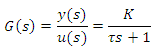125

Let the sinusoidal input u(t) = A sin ωt perturb the system. Then the output of the process will be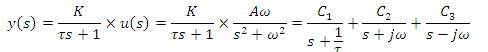Computing the constants C1,C2and C3 and taking inverse Laplace Transform of the above equation we obtain,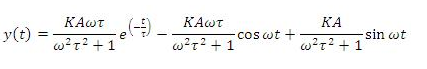127

After sufficiently long time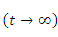, the first term disappears as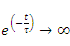Hence, using the identity eq. (91) we obtain,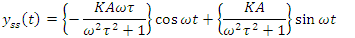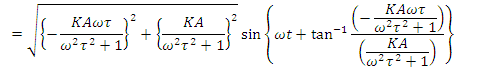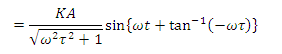128

Hence we observe that

•  Sinusoidal output wave has the same frequency as that of input sinusoid

•  Amplitude Ratio between the output wave and input wave is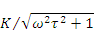•  Output wave lags behind the input wave with a phase difference of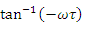Fig. III.13 shows the Input and Output wave profile for a frequency response analysis.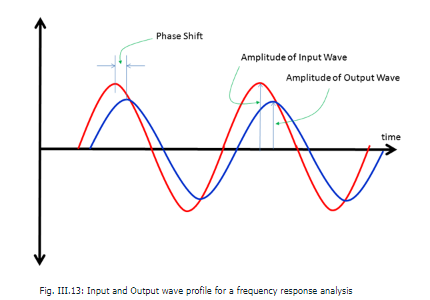Complex Plane and Frequency Response Analysis

Consider a complex number W = a + jb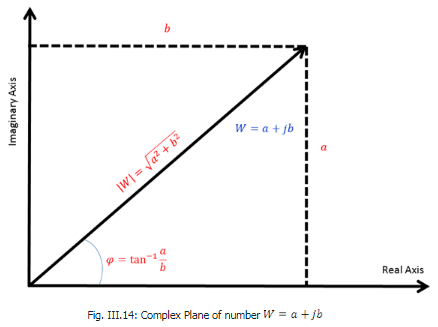The modulus (or absolute value or magnitude) of W is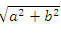and the argument (or phase angle) is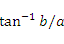. Let us put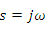in the transfer function of the first order process as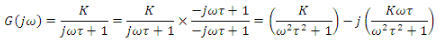129
As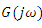is now a complex number, the modulus and argument can be calculated as,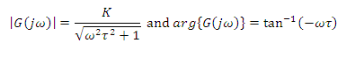130

The last two relationships indicate the amplitude ratio and phase lag for the ultimate response of the first order process. Hence the observations in the last subsection can also be stated in the light of the above results as follows:

•  Sinusoidal output wave has the same frequency as that of input sinusoid

•  Amplitude Ratio between the output wave and input wave is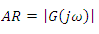•  Output wave lags behind the input wave with a phase difference of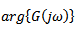Example of frequency response of a second order system
The process is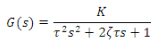131

Put and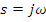calculate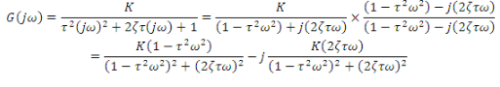132

then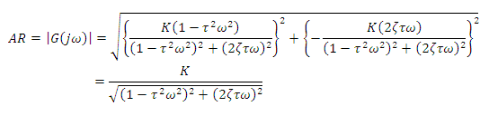133

and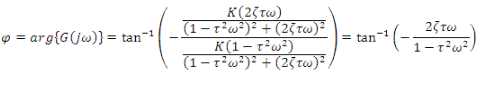134

Offer running on EduRev: Apply code STAYHOME200 to get INR 200 off on our premium plan EduRev Infinity!

,

,

,

,

,

,

,

,

,

,

,

,

,

,

,

,

,

,

,

,

,

;